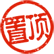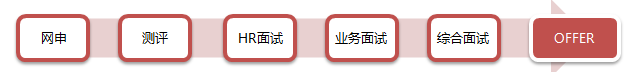1、营销管理类:http://job.fotile.com/xyzp?k=&c=-1&p=3^-1,1^9&day=-1&PageIndex=1&class=2#zw

2、研发技术类:http://job.fotile.com/xyzp?k=&c=-1&p=3^-1,1^11&day=-1&PageIndex=1&class=2#zw

3、供应链类（制造技术）:http://job.fotile.com/xyzp?k=&c=-1&p=3^-1,1^5&day=-1&PageIndex=1&class=2#zw

4、设计类:http://job.fotile.com/xyzp?k=&c=-1&p=3^-1,1^17&day=-1&PageIndex=1&class=2#zw

5、IT类:http://job.fotile.com/xyzp?k=&c=-1&p=3^-1,1^14&day=-1&PageIndex=1&class=2#zw

6、职能类:http://job.fotile.com/xyzp?k=&c=-1&p=3^-1,1^6&day=-1&PageIndex=1&class=2#zw

2020年12月―2021年10月期间于国内外院校毕业的2021届毕业生。

2016年和2018年，方太两度荣膺全球顶尖的人力资源咨询机构――怡安翰威特（Aon Hewitt）――评选的年度中国最佳雇主荣誉。最佳雇主之旅是您在职业生涯发展中不可多得的一份经历。1、电脑端：http://job.fotile.com，选择“校园招聘”，点击应聘岗位，在线网申。
2、手机端：微信扫描二维码，关注“FOTILE方太”，在线网申。

（保存二维码至手机，便于您查询应聘进度）• 浏览：924 评论：0 人